• 2020-09-05 05:37:00

时间序列指数平滑预测法

In this post, we describe and explain certain classical algorithms that forecast future values of time series. These algorithms, called exponential smoothers, have been in wide use for many decades. We convey intuition with examples, some augmented with Python code in an appendix

在这篇文章中，我们描述并解释了某些预测时间序列未来值的经典算法。 这些称为指数平滑器的算法已经广泛使用了数十年。 我们通过示例传达直觉，附录中还增加了一些Python代码

Exponential smoothers continue to remain attractive in the modern age of deep machine learning. They are simple and easy to implement. They are also surprisingly effective for short-term forecasting. Especially on rapidly-changing time series.

在深度机器学习的现代时代，指数平滑器继续保持吸引力。 它们简单易行。 它们对于短期预测也非常有效。 特别是在快速变化的时间序列上。

Exponential smoothers also lend themselves to incremental learning, often a must-have on rapidly-changing time series. This is so that the algorithm can keep up with the changing characteristics. Such as a trending time series suddenly starting to oscillate.

指数平滑器还适合进行增量学习，这通常是快速变化的时间序列的必备条件。 这样，算法就可以跟上变化的特征。 例如趋势时间序列突然开始振荡。

Basic Concepts

基本概念

A time series is a sequence of values over time. Such as daily average temperature in a city, the daily closing price of a particular stock, monthly sales of iPhone 11.

时间序列是一段时间内的一系列值。 例如城市的每日平均温度，特定股票的每日收盘价，iPhone 11的月销售量。

Our interest here is in forecasting future values of a time series from its historical values. (In a broader formulation, which we won’t cover here, we might include additional predictors, even additional time series.)

我们的兴趣是根据历史值预测时间序列的未来值。 (在更广泛的表述中，我们可能不会在此处介绍，我们可能会包括其他预测变量，甚至是其他时间序列。)

This problem is of immense interest. Businesses want to forecast future sales. Traders would like to forecast stock or index prices when possible. We all want to forecast the weather.

这个问题引起了极大的关注。 企业希望预测未来的销售。 交易者希望在可能的情况下预测股票或指数价格。 我们都想预测天气。

Next, a key concept. The forecast horizon, a positive integer h, is the number of steps ahead we’d like to forecast. So for a daily time series, a forecast horizon of 1 would forecast the next day’s value, a forecast horizon of 7 would forecast its value 7 days into the future.

接下来，是一个关键概念。 预测范围 (正整数h )是我们要预测的前进步数。 因此，对于每日时间序列，预测范围1预测第二天的值，预测范围7预测未来7天的值。

We start with the naive forecaster (NF). While it’s too naive to use, it serves as a useful baseline to compare against the various exponential smoothers.

我们从幼稚的预测器 (NF)开始。 虽然它太天真了，但可以用作与各种指数平滑器进行比较的有用基准。

The naive forecaster forecasts x(t+h) to be x(t). Note that, regardless of what h is, the forecast is always x(t).

天真的预报员将x ( t + h )预测为x ( t )。 注意，无论h是什么，预测始终为x ( t )。

Now, on to our first exponential smoother.

现在，进入我们的第一个指数平滑器。

Simple Exponential Smoothing (SES)

简单指数平滑(SES)

Let’s model our time series as follows:

让我们对时间序列建模如下：

x(t) = f(t) + noise

x (t)= f ( t )+噪声

Here f(t) is a deterministic function of t, and noise is independently generated at each time step by sampling from a suitable distribution, e.g. standard normal. This model is both rich and intuitively appealing. f(t) models the deterministic component of the time series. This can be pretty elaborate, including multiple trends and multiple seasonalities if we like. noise models random fluctuations and other unmodeled effects.

这里F(t)t的确定性函数，并且噪声被独立地通过从合适的分布采样在每个时间步骤中产生，例如标准正常。 该模型既丰富又直观。 f ( t )对时间序列的确定性分量建模。 如果我们愿意，这可能非常复杂，包括多个趋势和多个季节。 噪声对随机波动和其他未建模的影响进行建模。

Below are some examples

以下是一些例子

x(t) = t + noisex(t) = log(t) + noisex(t) = t² + noisex(t) = sin(t) + noise

The main idea in SES is to estimate f(t) from x(t), x(t-1), …, and then use this estimate to forecast future values of x.

SES的主要思想是从x ( t )， x ( t -1)，…估计f ( t )，然后使用该估计值预测x的未来值。

SES’s estimate of f(t) is the exponentially-weighted average of x(t), x(t-1), x(t-2), etc. x(t) contributes the most, x(t-1) the second most, x(t-2) the third most, and so on. The contributions decay exponentially, at a rate controlled by a decay parameter a.

SES对f ( t )的估计是x ( t )， x ( t -1)， x ( t -2)等的指数加权平均值。x ( t )贡献最大， x ( t -1)第二大， x ( t -2)第三大，依此类推。 贡献以由衰减参数a控制的速率呈指数衰减。

This may be expressed recursively as

这可以递归表示为

f^(t) = ax(t) + (1-a)f^(t)

f ^( t )= ax ( t )+(1- a ) f ^( t )

The ^ is there to remind us that this is an estimate of f. Not f itself, which is hidden from us.

^提醒我们这是f的估计 不是f本身，它对我们是隐藏的。

The forecast now is simply x^(t+h) = f^(t). NF is a special case of SES in which f^(t) = x(t).

现在的预测就是x ^( t + h )= f ^( t )。 NF是SES的一种特殊情况，其中f ^( t )= x ( t )。

For its simplicity, SES works surprisingly well for 1-step forecasts, i.e. h=1. The reason for this is that SES not only smooths out noise, but it also fits f(t) locally, i.e. at time t, which is important when the time series is changing rapidly. NF also fits f(t) locally, however, it does not smooth out noise. SES is ineffective for longer-term forecasts, i.e. h > 1.

为了简单起见，SES对于1步预测(即h = 1)出奇地好。 这是因为SES不仅可以消除噪声，而且还可以局部拟合f ( t )，即在时间t处 ，这在时间序列快速变化时很重要。 NF也可以局部拟合f ( t )，但是不能消除噪声。 SES对于长期预测无效，即h > 1。

Simple Exponential Smoothing + Trend (TrES)

简单指数平滑+趋势(TrES)

As mentioned earlier, SES is ineffective for longer-term forecasts. By adding a trending component to SES, we can improve the situation. The intuition is simple. If there is a local trend at x(t), a trend component can estimate it, and use it to forecast for somewhat longer horizons. (This is similar to the reasoning: if you know an object’s current position and velocity, you can predict where it will be a little later.)

如前所述，SES对于长期预测无效。 通过向SES添加趋势组件，我们可以改善这种情况。 直觉很简单。 如果在x ( t )处有局部趋势，则趋势分量可以对其进行估计，并使用它来预测更长的时间范围。 (这与推理类似：如果您知道对象的当前位置和速度，则可以预测稍后的位置。)

Here is a simple example. Consider x(t) = t + noise. Say somehow we figure out that x(t) is growing by 1 in every time unit (noise aside). Knowing this we can forecast that x’s value h time units later will be its current value plus h.

这是一个简单的例子。 考虑x ( t )= t +噪声。 可以说，我们发现x ( t )在每个时间单位(除噪声)中均增长1。 知道了这一点，我们可以预测x的值h时间单位将是其当前值加h

Okay, let’s describe the algorithm more thoroughly. For this, let’s reexpress our time series as

好的，让我们更全面地描述算法。 为此，让我们将时间序列重新表示为

x(t) = f(t-1) + df(t-1) + noise

x ( t )= f ( t -1)+ df ( t -1)+噪声

Here f(t’) is a function of t’ and df(t’) is the local trend at time t’. We have written it as df(t’) because it is the discrete analog of the first derivative of f at t’.

这里F(T“)t的函数”和df(T“)是在时间t的本地趋势”。 我们将其写为df ( t ')，因为它是ft '时的一阶导数的离散模拟。

This model is equivalent to our first model. We’ve just factored f(t) as f(t-1) + df(t-1).

此模型等效于我们的第一个模型。 我们只是将f ( t )分解为f ( t -1)+ df ( t -1)。

Below are some refactored examples.

以下是一些重构示例。

x(t) = (t-1) + 1 + noisex(t) = (t-1)² + 2t + noise

What’s the point of this factoring? We can estimate the two terms f(t-1) and df(t-1) separately. This allows us to make sensible longer term forecasts on series in which df(t) can be accurately estimated. Such as in x(t) = t + noise. Under the factoring x(t) = (t-1) + 1 + noise we see that df(t) equals 1. Using this estimate lets us make sensible forecasts further out into the future.

这个分解的意义是什么？ 我们可以分别估计两个项f ( t -1)和df ( t -1)。 这使我们能够对可以准确估计df ( t )的序列进行合理的长期预测。 如x ( t )= t +噪声。 在因子x ( t )=( t -1)+1 +噪声下，我们看到df ( t )等于1。使用此估计值，我们可以对未来做出更明智的预测。

How do we estimate f(t-1) and df(t-1)? Both via exponential smoothing. f(t)’s estimate is the exponentially-weighted average of x(t), x(t-1), …. df(t)’s estimate is the exponentially-weighted average of x(t) - x(t-1), x(t-1) - x(t-2), x(t-2) - x(t-3), …

我们如何估计f ( t -1)和df ( t -1)？ 两者都通过指数平滑。 f ( t )的估计值是x ( t )， x ( t -1)，…的指数加权平均值。 df ( t )的估计值是x ( t ) -x ( t -1)， x ( t -1) -x ( t -2)， x ( t -2) -x ( t的指数加权平均值-3)，...

Let’s call these estimates f^(t) and df^(t) respectively. The forecast is now

让我们分别称这些估计为f ^( t )和df ^( t )。 现在是预报

x^(t+h) = f^(t) + h*df^(t)

x ^( t + h )= f ^( t )+ h * df ^( t )

Note that f^(t) and df^(t) are both local estimates. So the algorithm adapts to changes in f(t) as well as df(t). We can use a and b as the exponential-decay parameters for estimating f and df respectively. This gives us more knobs to tweak. (Following the example below, we will discuss tuning these knobs a bit.)

注意， f ^( t )和df ^( t )都是局部估计。 因此，该算法可以适应f ( t )和df ( t )的变化。 我们可以使用ab作为指数衰减参数来分别估计fdf 。 这为我们提供了更多调整的旋钮。 (在下面的示例中，我们将讨论如何微调这些旋钮。)

Example

t           1   2    3    4    5     ...x           1   2    3    4    5     ...f^(a=½)     2   2.5  3.25 ... df^(b=½)    1   1    1    ...

f^(2) is 2 because we have initialized it to x(2). df^(2) is 1 because we have initialized it to x(2)-x(1). We see that the algorithm has deduced the trend that the series grows by 1 in every time step.

f ^(2)为2，因为我们已将其初始化为x (2)。 df ^(2)为1，因为我们已将其初始化为x (2) -x (1)。 我们看到，该算法推导了序列在每个时间步长增长1的趋势。

Let’s calculate x^(t+1) at time t=4. It is f^(4) + df^(4) = 3.25 + 1 = 4.25. Close to the actual value x(5) = 5 though lagging a bit. The lag is a consequence of the exponential smoothing. We could reduce the lag by weighing recency higher, but that may incur a cost elsewhere. We discuss trade-offs involving this in the next paragraph.

让我们计算在时间t = 4时x ^( t +1)。 它是f ^(4)+ df ^(4)= 3.25 + 1 = 4.25。 尽管有点滞后，但接近实际值x(5)= 5。 滞后是指数平滑的结果。 我们可以通过增加新近度来减少滞后，但这可能会导致其他方面的成本。 我们将在下一段中讨论与此相关的取舍。

Next, let’s calculate x^(t+3) at time t = 4. It is f^(4) + 3*df^(4) = 3.25 + 3*1 = 6.25. Clearly we have been able to exploit the trend to forecast further into the future! x(7) is 7. The longer horizon forecast is as accurate as the 1-step one in this case! The lag hasn’t increased.

接下来，让我们在时间t = 4处计算x ^( t +3)。它是f ^(4)+ 3 * df ^(4)= 3.25 + 3 * 1 = 6.25。 显然，我们已经能够利用这一趋势来进一步预测未来！ x(7)为7。在这种情况下，较长的地平线预测与1步预测一样准确！ 滞后并没有增加。

The smoothing parameters offer us ways to control the lags. In our example, increasing the value of a will reduce the lag. On the other hand, on a noisy version of our example, an overly large value of a will not be able to smooth out the noise. So yes, the lag will reduce, but not the forecast quality. It will be as if we are chasing the most recent value, moving with the noise in it.

平滑参数为我们提供了控制滞后的方法。 在我们的例子中，增加价值将减少滞后。 另一方面，在我们示例的嘈杂版本中，太大的a值将无法消除噪声。 所以是的，延迟会减少，但预测质量不会减少。 好像我们正在追赶最新的价值，随着其中的噪音而移动。

Similar reasoning applies to the parameter b. Except that it applies to df, i.e. to the slope. In our example, the slope was always the same, 1. So changing b would not have any effect. However, if the slope was greater than 1 and had multiplicative noise in it, e.g. df was 5*noise where noise has a mean of 1 and fluctuates around it, changing b would likely have an impact on how much df^ lags df versus how much df^ chases the noise in df.

类似的推理适用于参数b 。 除了适用于df ，即斜率。 在我们的示例中，斜率始终为1。因此，更改b不会产生任何影响。 但是，如果斜率大于1且其中具有乘性噪声，例如df为5 *噪声，其中噪声的平均值为1并在其附近波动，则更改b可能会影响df ^滞后df与如何多DF ^追逐在DF的噪音。

The way to go here is to auto-tune these parameters on suitable slices of the input data. That is, machine-learn them to minimize a suitable loss function. We won’t go into the details here.

此处的方法是在输入数据的适当片段上自动调整这些参数。 即，机器学习它们以最小化合适的损失函数。 我们将不在这里详细介绍。

Simple Exponential Smoothing + Trend + Seasonality (TrSeES)

简单指数平滑+趋势+季节性(TrSeES)

SeES, while effective on series that exhibit locally linear trends, is not always effective on series with cyclic structure.

看到 对表现出局部线性趋势的序列有效，对具有循环结构的序列并不总是有效。

Consider the time series

考虑时间序列

1, 2, 3, 1, 2, 3, 1, 2, 3, … 

SeES will predict x^(3) to be close to 4. In reality, x(3) is 1. That’s a big difference. If we somehow knew that the time series repeats itself every three time steps we could use this information to improve the forecast.

SeES将预测x ^(3)接近4。实际上， x (3)为1。这是一个很大的差异。 如果我们以某种方式知道时间序列每三个时间步重复一次，则可以使用此信息来改进预测。

How can we improve the TrES? Let’s assume the series has a single seasonality, i.e. a single repeating component, and we know its order, i.e. k. We can model such a time series as follows.

我们如何改善TrES？ 假设该系列具有单个季节性，即单个重复分量，我们知道其顺序，即k 。 我们可以对这样的时间序列建模如下。

x(t) = tr(t) + s(t) + noise

x ( t )= tr ( t )+ s ( t )+噪声

s(t) = s(t-k)

s ( t )= s ( t - k )

Here s(t) is a repeating time series. x(t) is obtained by adding s(t) to another time series which we are calling tr(t). We are calling it tr(t) because we think of it as modeling x(t)’s trend component. That said, in reality, tr(t) models whatever is left over after removing s(t) from x(t). It may yet have other repeating components. tr(t) is just less seasonable than x(t). As will become clear when we see the algorithm below, even removing a single seasonality will be progress.

s ( t )是重复的时间序列。 X(t)是通过将S(t) ，这是我们呼吁TR(t)另一时间序列获得。 之所以称其为tr ( t )，是因为我们将其视为建模x ( t )的趋势分量。 就是说，实际上， tr ( t )对从x ( t )除去s ( t )后剩下的任何东西进行建模。 它可能还具有其他重复组件。 tr ( t )与x ( t )相比，季节性更差。 正如我们在下面的算法中看到的那样，即使去除单个季节性也将是进步。

Let’s see an example expressed this way.

让我们来看一个以这种方式表达的示例。

t   1  2  3  4  5  6  7  8  9s   1  2  3  1  2  3  1  2  3tr  1  2  3  4  5  6  7  8  9x   2  4  6  5  7  9  8  10 12

How do we estimate tr(t) and s(t)? First, we assume we know the order k. One sensible approach is to obtain a new time series yk(t) = x(t) - x(t - k) with the influence of the seasonality removed. We can then apply TrES to forecast yk^(t+h). We then add x(t+h - k) to yk^(t+h) which gives us a sensible forecast x^(t+h) of x(t + h).

我们如何估计tr ( t )和s ( t )？ 首先，我们假设我们知道阶k 。 一种明智的方法是在去除季节性影响的情况下获得新的时间序列yk ( t )= x ( t ) -x ( t - k )。 然后，我们可以将TrES应用于预测yk ^( t + h )。 然后，我们添加X(T + H - K)YK ^(T + H)，这给了我们x的合理预测的x ^(T + H)(T + H)。

Let’s see how this plays out in the example above.

让我们看看上面的示例中的情况。

t   1  2  3  4  5  6  7  8  9x   2  4  6  5  7  9  8  10 12y3  3  3  3  3  3  3

Great, y3 is easy to forecast on. Its value is always 3. Removing the seasonality’s influence helped a lot! TrES will quickly figure out that y3 is constant.

很好，y3很容易预测。 它的值始终为3。消除季节性因素的影响很大！ TrES会很快确定y3是常数。

Let’s forecast x(t+3=12) at t=9. First we forecast y3(12) to be 3. Then we add x(9) = 12 to this forecast. We get x^(12) = 3+12 = 15. This forecast is accurate unless the future changes course.

让我们在t = 9时预测x ( t + 3 = 12)。 首先，我们将y 3(12)预测为3。然后将x (9)= 12添加到该预测中。 我们得到x ^(12)= 3 + 12 =15。除非将来改变路线，否则此预测是准确的。

We can do this so long as h is less than or equal to k. (If h were greater than k, t+h - k would be greater than t, i.e. into the future!)

只要h小于或等于k，我们就可以这样做。 (如果h大于k ，则t + h - k将大于t ，即到将来！)

Smoothing The Seasonality

平滑季节性

We can improve on TrSeES further. In deriving x^(t+h) from y^k(t+h) instead of adding x(t+h - k) we can add a smoothed version of it. Smoothed in what way? By taking the exponentially-weighted average of x(t+h - k), x(t+h - 2k), x(t+h - 3k), …

我们可以进一步改善TrSeES。 从y ^ k ( t + h )导出x ^( t + h )而不是添加x ( t + h - k )时，我们可以添加它的平滑版本。 用什么方式平滑？ 通过取x ( t + h - k )， x ( t + h -2 k )， x ( t + h -3 k )的指数加权平均值，…

Why do we think smoothing the seasonality improves TrSeES further? Consider the case when the seasonality component has multiplicative noise in it. I.e.,

为什么我们认为平滑季节性可以进一步改善TrSeES？ 考虑当季节性分量中包含乘性噪声的情况。 即

s(t) = noise*s(t-k)

s ( t )=噪声* s ( t - k )

where, for illustration purposes, let’s say this noise is a Gaussian with a mean of 1 and a standard deviation greater than 0 but much less than 1. Such a seasonality model is realistic.

在这里，出于说明目的，假设此噪声是高斯噪声，平均值为1，标准偏差大于0但远小于1。这样的季节性模型是现实的。

In this case, x(t+h - k), x(t+h - 2k), x(t+h - 3k), … will also be influenced by such multiplicative noise. TrES will address the additive noise we previously had. But not this multiplicative noise. Using an exponentially-smoothed estimate of x(t+h - k) from x(t+h - k), x(t+h - 2k), x(t+h - 3k), …, rather than x(t+h - k) will help alleviate the effect of this noise on the forecast.

在这种情况下， x ( t + h - k )，x( t + h -2 k )， x ( t + h -3 k )，…也会受到这种乘法噪声的影响。 TrES将解决我们以前遇到的附加噪声。 但不是这种乘性噪声。 用x的指数平滑估计(T + H - k)的X(t + H - K)，X(T + H - 2 K)，X(T + H - 3 K)，...，而大于x ( t + h-k )将有助于减轻这种噪声对预报的影响。

Python Code

Python代码

I included it here so people can see some actual code. That said, it’s incomplete in parts. There might also be bugs. So be prepared to do some additional work if you want to get it all working.

我将其包含在此处，以便人们可以看到一些实际代码。 就是说，它部分不完整。 可能还会有错误。 因此，如果您想使其全部工作，请准备做一些额外的工作。

Time series generator

时间序列发生器

import numpy as npnoise = np.random.normalf = lambda t: <some function of t>x = [f(t) + noise for t in range(n)]

By choosing f suitably we can generate a variety of time series. Including ones with trends, ones with cycles, and ones with both.

通过适当选择f，我们可以生成各种时间序列。 包括具有趋势的，具有周期的，以及两者兼有的。

Next, we show the code for the various exponential smoothers. As the first statement in all of them, add

接下来，我们显示各种指数平滑器的代码。 作为所有这些中的第一条陈述，添加

x = [1,2,3,4,5,6,7]

Or, better, use the time series generator to generate x.

或者，最好使用时间序列生成器生成x。

SES algorithm

SES算法

<initialize x here>f = xy = {}a = 0.5for t in range(len(x)):   xhat_tplus1 = f        # forecast next value   f = a*x[t] + (1-a)*f

TrES algorithm

TrES算法

<initialize x here>f,df    = x, x-xy       = {}h, a, b = 3, 0.5, 0.5for t in range(2,len(x)):   x_hat_tplush = f + h*df # forecast h steps ahead   f  = a*x[t] + (1-a)*f   df = b*(x[t]-x[t-1]) + (1-b)*df

TrSeES

交易

This needs more work to get it going end-to-end. It also does not smoothen the seasonality.

这需要更多的工作才能使其端到端地运行。 这也不会使季节性变得平滑。

<initialize x here>yk = [x[t] — x[t-k] for t in range(k,n)]<Run TrES on yk># Forecast x. forecast_y(t+h) uses the forecast model built earlier on yk using TrES.xhat_tplus_h = forecast_y(t+h) + x[t+h-k]

进一步阅读

1. https://otexts.com/fpp2/ Chap 7 is on exponential smoothing.

https://otexts.com/fpp2/第7章介绍了指数平滑。

时间序列指数平滑预测法python 人工智能 机器学习 java 算法
更多相关内容
•C++
• ## 指数平滑方法简介

千次阅读 2021-02-04 02:26:43
指数平滑(Exponential smoothing)是除了 ARIMA 之外的另一种被广泛使用的时间序列预测方法(关于 ARIMA，请参考 时间序列模型简介)。 指数平滑即指数移动平均(exponential moving average)，是以指数式递减加权的移动...

本文链接：个人站 | 简书 | CSDN

版权声明：除特别声明外，本博客文章均采用 BY-NC-SA 许可协议。转载请注明出处。

指数平滑(Exponential smoothing)是除了 ARIMA 之外的另一种被广泛使用的时间序列预测方法(关于 ARIMA，请参考 时间序列模型简介)。 指数平滑即指数移动平均(exponential moving average)，是以指数式递减加权的移动平均。各数值的权重随时间指数式递减，越近期的数据权重越高。常用的指数平滑方法有一次指数平滑、二次指数平滑和三次指数平滑。

1. 一次指数平滑

一次指数平滑又叫简单指数平滑(simple exponential smoothing， SES)，适合用来预测没有明显趋势和季节性的时间序列。其预测结果是一条水平的直线。模型形如：

Forecast equation:Smoothing equantion:其中是真实值，为预测值，为平滑值，定义残差，其中，则可以通过优化方法得到使用 python 的 statsmodels 可以方便地应用该模型：

import numpy as np

import pandas as pd

import matplotlib.pyplot as plt

from statsmodels.tsa.holtwinters import SimpleExpSmoothing

x1 = np.linspace(0, 1, 100)

y1 = pd.Series(np.multiply(x1, (x1 - 0.5)) + np.random.randn(100))

ets1 = SimpleExpSmoothing(y1)

r1 = ets1.fit()

pred1 = r1.predict(start=len(y1), end=len(y1) + len(y1)//2)

pd.DataFrame({

'origin': y1,

'fitted': r1.fittedvalues,

'pred': pred1

}).plot(legend=True)

效果如图：ses.png

2. 二次指数平滑

2.1 Holt's linear trend method

Holt 扩展了简单指数平滑，使其可以用来预测带有趋势的时间序列。直观地看，就是对平滑值的一阶差分(可以理解为斜率)也作一次平滑。模型的预测结果是一条斜率不为0的直线。模型形如：

Forecast equation:Level equation:Trend equation:其中import numpy as np

import pandas as pd

import matplotlib.pyplot as plt

from statsmodels.tsa.holtwinters import Holt

x2 = np.linspace(0, 99, 100)

y2 = pd.Series(0.1 * x2 + 2 * np.random.randn(100))

ets2 = Holt(y2)

r2 = ets2.fit()

pred2 = r2.predict(start=len(y2), end=len(y2) + len(y2)//2)

pd.DataFrame({

'origin': y2,

'fitted': r2.fittedvalues,

'pred': pred2

}).plot(legend=True)

效果如图：holt.png

2.2 Damped trend methods

Holt's linear trend method 得到的预测结果是一条直线，即认为未来的趋势是固定的。对于短期有趋势、长期趋于稳定的序列，可以引入一个阻尼系数，将模型改写为

Forecast equation:Level equation:Trend equation:3. 三次指数平滑

为了描述时间序列的季节性，Holt 和 Winters 进一步扩展了 Holt's linear trend method，得到了三次指数平滑模型，也就是通常说的 Holt-Winters’ 模型。我们用表示“季节”的周期。根据季节部分和非季节部分的组合方式不同，Holt-Winters’ 又可以分为加法模型和乘法模型。

加法模型形如：

Forecast equation:Level equation:Trend equation:Seasonal equation:其中的整数部分。

import numpy as np

import pandas as pd

import matplotlib.pyplot as plt

from statsmodels.tsa.holtwinters import ExponentialSmoothing

x3 = np.linspace(0, 4 * np.pi, 100)

y3 = pd.Series(20 + 0.1 * np.multiply(x3, x3) + 8 * np.cos(2 * x3) + 2 * np.random.randn(100))

r3 = ets3.fit()

pred3 = r3.predict(start=len(y3), end=len(y3) + len(y3)//2)

pd.DataFrame({

'origin': y3,

'fitted': r3.fittedvalues,

'pred': pred3

}).plot(legend=True)

效果如图：3.2 Holt-Winters’ multiplicative method

乘法模型形如：

Forecast equation:Level equation:Trend equation:Seasonal equation:效果如图：holt_winters_mul.png

3.3 Holt-Winters’ damped method

Holt-Winters’ 模型的趋势部分同样可以引入阻尼系数，这里不再赘述。

4. 参数优化和模型选择

参数优化的方法是最小化误差平方和或最大化似然函数。模型选择可以根据信息量准则，常用的有 AIC 和 BIC等。

AIC 即 Akaike information criterion， 定义为其中是似然函数，是参数数量。用 AIC 选择模型时要求似然函数大，同时对参数数量作了惩罚，在似然函数相近的情况下选择复杂度低的模型。

BIC 即 Bayesian information criterion，定义为其中是样本数量。当时，，因此当样本量较大时 BIC 对模型复杂度的惩罚比 AIC 更严厉。

5. 与 ARIMA 的关系

线性的指数平滑方法可以看作是 ARIMA 的特例。例如简单指数平滑等价于 ARIMA(0, 1, 1)，Holt's linear trend method 等价于 ARIMA(0, 2, 2)，而 Damped trend methods 等价于 ARIMA(1, 1, 2) 等。

我们不妨来验证一下。可以改写为亦即两边同时加上，得而 ARIMA(p, d, q) 可以表示为其中是滞后算子(Lag operator)，考虑 ARIMA(0, 1, 1)亦即，则两者等价。

非线性的指数平滑方法则没有对应的 ARIMA 表示。

参考文献

 Hyndman, Rob J., and George Athanasopoulos. Forecasting: principles and practice. OTexts, 2014.

 Exponential smoothing - Wikipedia https://en.wikipedia.org/wiki/Exponential_smoothing

 Introduction to ARIMA models - Duke https://people.duke.edu/~rnau/411arim.htm

展开全文•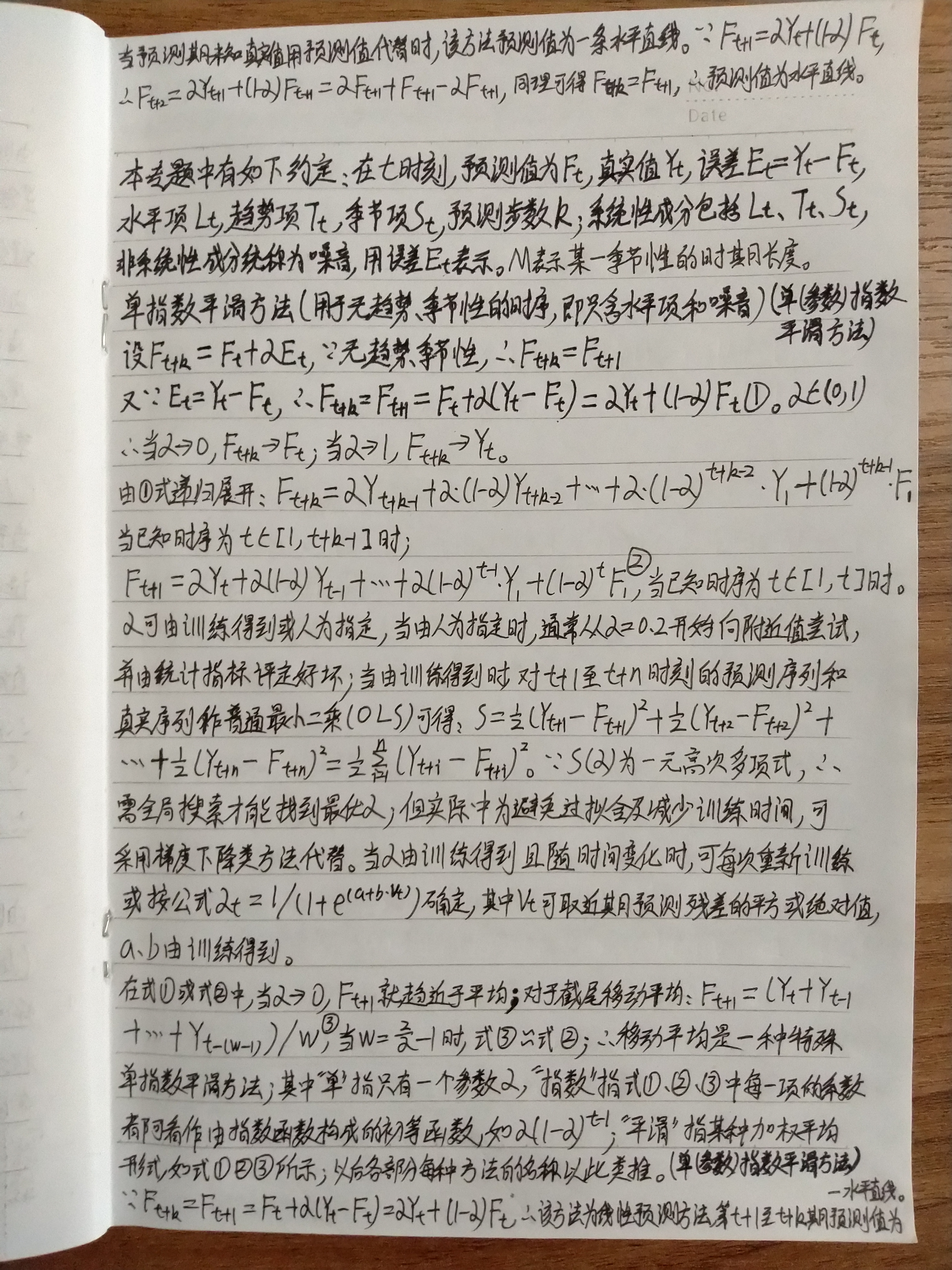展开全文时间序列预测
• 文章目录0、特别说明0.1 参考来源0.2 包版本号1、简介2、一次指数平滑2.1 理论介绍2.2 代码展示2.3 参数介绍3、 二次指数平滑3.1 理论介绍3.1.1 Holt’s linear trend method3.1.2 Damped trend methods3.2 代码展示...

@创建于：20210324
@修改于：20210324

### 特别说明

#### 参考来源

本文理论内容转自下面三个博客，它们为同一位作者。代码部分我做了改动。

#### 包版本号

本文测试所用的版本号：

• python 3.8.5
• statsmodels 0.12.2
• pandas 1.2.2

### 1、简介

指数平滑（Exponential smoothing）是除了 ARIMA 之外的另一种被广泛使用的时间序列预测方法。 指数平滑即指数移动平均（exponential moving average），是以指数式递减加权的移动平均。各数值的权重随时间指数式递减，越近期的数据权重越高。常用的指数平滑方法有一次指数平滑、二次指数平滑和三次指数平滑。

### 2、一次指数平滑

#### 2.1 理论介绍

一次指数平滑又叫简单指数平滑（simple exponential smoothing， SES），适合用来预测没有明显趋势和季节性的时间序列。其预测结果是一条水平的直线。模型形如：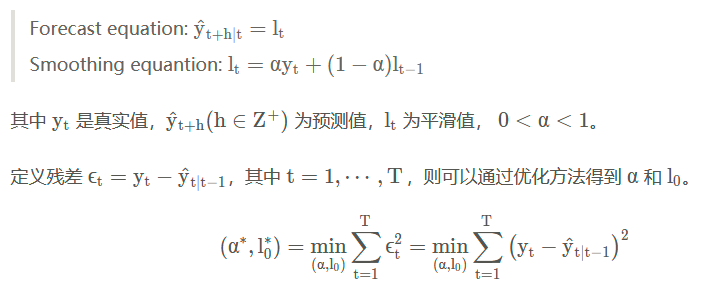#### 2.2 代码展示

使用 python 的 statsmodels 可以方便地应用该模型：

import numpy as np
import pandas as pd
import matplotlib.pyplot as plt

def ses():
from statsmodels.tsa.holtwinters import SimpleExpSmoothing
number = 30
x1 = np.round(np.linspace(0, 1, number), 4)
y1 = pd.Series(np.multiply(x1, (x1 - 0.5)) + np.random.randn(number))
# fitted部分是直线或者是曲线，受到原始数据影响。
# 多次测试显示，直线的概率高。
# ets1 = SimpleExpSmoothing(endog=y1, initialization_method='estimated')
ets1 = SimpleExpSmoothing(endog=y1, initialization_method='heuristic')
r1 = ets1.fit()
pred1 = r1.predict(start=len(y1), end=len(y1) + len(y1)//2)

pd.DataFrame({
'origin': y1,
'fitted': r1.fittedvalues,
'pred': pred1
}).plot()
plt.savefig('ses.png')

ses()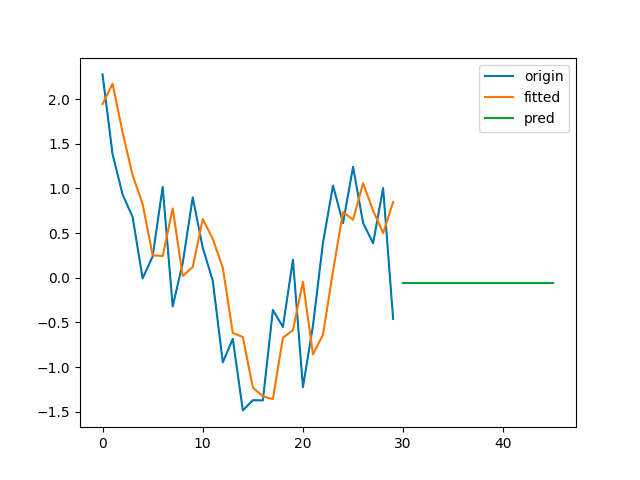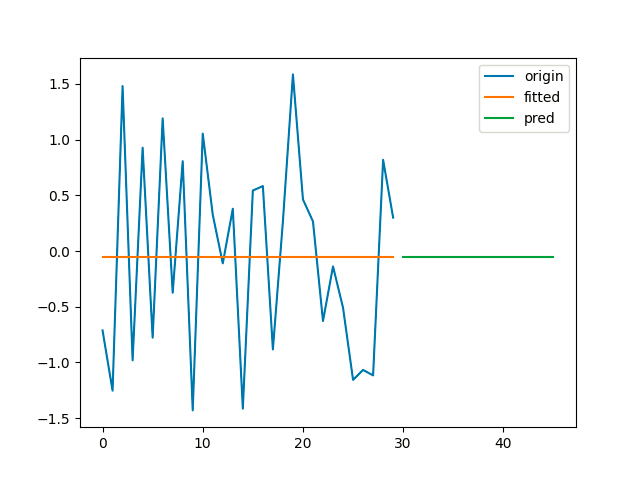#### 2.3 参数介绍

Simple Exponential Smoothing
Parameters
----------
endog : array_like
The time series to model.
initialization_method : str, optional
Method for initialize the recursions. One of:

* None
* 'estimated'
* 'heuristic'
* 'legacy-heuristic'
* 'known'

None defaults to the pre-0.12 behavior where initial values
are passed as part of fit. If any of the other values are
passed, then the initial values must also be set when constructing
the model. If 'known' initialization is used, then initial_level
must be passed, as well as initial_trend and initial_seasonal if
applicable. Default is 'estimated'. "legacy-heuristic" uses the same
values that were used in statsmodels 0.11 and earlier.
initial_level : float, optional
The initial level component. Required if estimation method is "known".
If set using either "estimated" or "heuristic" this value is used.
This allows one or more of the initial values to be set while
deferring to the heuristic for others or estimating the unset
parameters.

Fit the model

Parameters
----------
smoothing_level : float, optional
The smoothing_level value of the simple exponential smoothing, if
the value is set then this value will be used as the value.
optimized : bool, optional
Estimate model parameters by maximizing the log-likelihood.
start_params : ndarray, optional
Starting values to used when optimizing the fit.  If not provided,
starting values are determined using a combination of grid search
and reasonable values based on the initial values of the data.
initial_level : float, optional
Value to use when initializing the fitted level.
use_brute : bool, optional
Search for good starting values using a brute force (grid)
optimizer. If False, a naive set of starting values is used.
use_boxcox : {True, False, 'log', float}, optional
Should the Box-Cox transform be applied to the data first? If 'log'
then apply the log. If float then use the value as lambda.
remove_bias : bool, optional
Remove bias from forecast values and fitted values by enforcing
that the average residual is equal to zero.
method : str, default "L-BFGS-B"
The minimizer used. Valid options are "L-BFGS-B" (default), "TNC",
"SLSQP", "Powell", "trust-constr", "basinhopping" (also "bh") and
"least_squares" (also "ls"). basinhopping tries multiple starting
values in an attempt to find a global minimizer in non-convex
problems, and so is slower than the others.
minimize_kwargs : dict[str, Any]
A dictionary of keyword arguments passed to SciPy's minimize
function if method is one of "L-BFGS-B" (default), "TNC",
"SLSQP", "Powell", or "trust-constr", or SciPy's basinhopping
or least_squares. The valid keywords are optimizer specific.
Consult SciPy's documentation for the full set of options.

Returns
-------
HoltWintersResults
See statsmodels.tsa.holtwinters.HoltWintersResults.


### 3、 二次指数平滑

#### 3.1 理论介绍

##### 3.1.1 Holt’s linear trend method

Holt 扩展了简单指数平滑，使其可以用来预测带有趋势的时间序列。直观地看，就是对平滑值的一阶差分（可以理解为斜率）也作一次平滑。模型的预测结果是一条斜率不为0的直线。模型形如：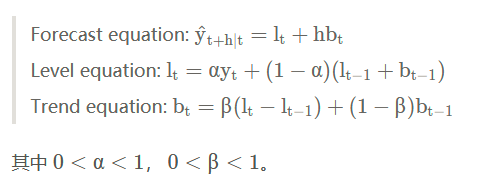##### 3.1.2 Damped trend methods

Holt’s linear trend method 得到的预测结果是一条直线，即认为未来的趋势是固定的。对于短期有趋势、长期趋于稳定的序列，可以引入一个阻尼系数 0<ϕ<1，将模型改写为：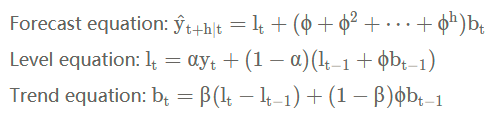#### 3.2 代码展示

import numpy as np
import pandas as pd
import matplotlib.pyplot as plt

def holt():
from statsmodels.tsa.holtwinters import Holt
number = 50
x2 = np.round(np.linspace(0, 99, number))
y2 = pd.Series(0.1 * x2 + 2 * np.random.randn(number))
# fitted部分是直线或者是曲线，受到原始数据影响。
# 多次测试显示，直线的概率高。
ets2 = Holt(endog=y2, initialization_method='estimated')
# ets2 = Holt(endog=y2, initialization_method='heuristic')
# ets2 = Holt(endog=y2, initialization_method='estimated', damped_trend=True)
r2 = ets2.fit()
pred2 = r2.predict(start=len(y2), end=len(y2) + len(y2) // 2)

pd.DataFrame({
'origin': y2,
'fitted': r2.fittedvalues,
'pred': pred2
}).plot(legend=True)
plt.savefig('holt2.png')

holt()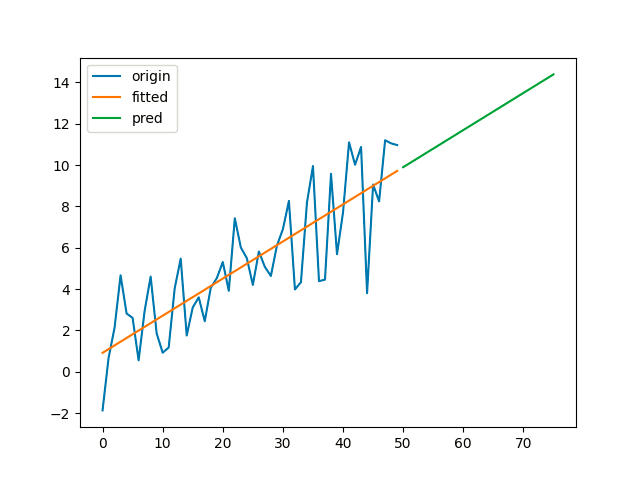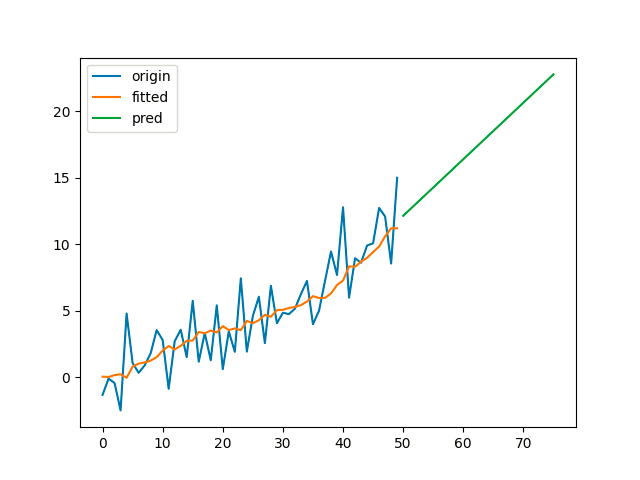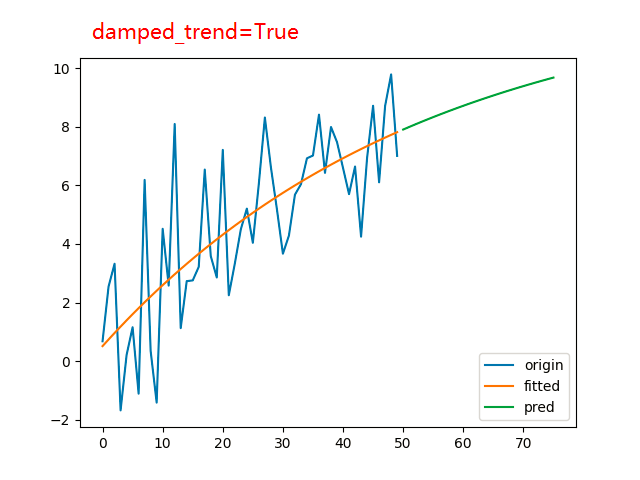#### 3.3 参数介绍

Holt's Exponential Smoothing

Parameters
----------
endog : array_like
The time series to model.
exponential : bool, optional
Type of trend component.
damped_trend : bool, optional
Should the trend component be damped.
initialization_method : str, optional
Method for initialize the recursions. One of:

* None
* 'estimated'
* 'heuristic'
* 'legacy-heuristic'
* 'known'

None defaults to the pre-0.12 behavior where initial values
are passed as part of fit. If any of the other values are
passed, then the initial values must also be set when constructing
the model. If 'known' initialization is used, then initial_level
must be passed, as well as initial_trend and initial_seasonal if
applicable. Default is 'estimated'. "legacy-heuristic" uses the same
values that were used in statsmodels 0.11 and earlier.
initial_level : float, optional
The initial level component. Required if estimation method is "known".
If set using either "estimated" or "heuristic" this value is used.
This allows one or more of the initial values to be set while
deferring to the heuristic for others or estimating the unset
parameters.
initial_trend : float, optional
The initial trend component. Required if estimation method is "known".
If set using either "estimated" or "heuristic" this value is used.
This allows one or more of the initial values to be set while
deferring to the heuristic for others or estimating the unset
parameters.

Fit the model

Parameters
----------
smoothing_level : float, optional
The alpha value of the simple exponential smoothing, if the value
is set then this value will be used as the value.
smoothing_trend :  float, optional
The beta value of the Holt's trend method, if the value is
set then this value will be used as the value.
damping_trend : float, optional
The phi value of the damped method, if the value is
set then this value will be used as the value.
optimized : bool, optional
Estimate model parameters by maximizing the log-likelihood.
start_params : ndarray, optional
Starting values to used when optimizing the fit.  If not provided,
starting values are determined using a combination of grid search
and reasonable values based on the initial values of the data.
initial_level : float, optional
Value to use when initializing the fitted level.

.. deprecated:: 0.12

Set initial_level when constructing the model

initial_trend : float, optional
Value to use when initializing the fitted trend.

.. deprecated:: 0.12

Set initial_trend when constructing the model

use_brute : bool, optional
Search for good starting values using a brute force (grid)
optimizer. If False, a naive set of starting values is used.
use_boxcox : {True, False, 'log', float}, optional
Should the Box-Cox transform be applied to the data first? If 'log'
then apply the log. If float then use the value as lambda.
remove_bias : bool, optional
Remove bias from forecast values and fitted values by enforcing
that the average residual is equal to zero.
method : str, default "L-BFGS-B"
The minimizer used. Valid options are "L-BFGS-B" (default), "TNC",
"SLSQP", "Powell", "trust-constr", "basinhopping" (also "bh") and
"least_squares" (also "ls"). basinhopping tries multiple starting
values in an attempt to find a global minimizer in non-convex
problems, and so is slower than the others.
minimize_kwargs : dict[str, Any]
A dictionary of keyword arguments passed to SciPy's minimize
function if method is one of "L-BFGS-B" (default), "TNC",
"SLSQP", "Powell", or "trust-constr", or SciPy's basinhopping
or least_squares. The valid keywords are optimizer specific.
Consult SciPy's documentation for the full set of options.

Returns
-------
HoltWintersResults
See statsmodels.tsa.holtwinters.HoltWintersResults.


### 4、 三次指数平滑

#### 4.1 理论介绍

为了描述时间序列的季节性，Holt 和 Winters 进一步扩展了 Holt’s linear trend method，得到了三次指数平滑模型，也就是通常说的 Holt-Winters’ 模型。我们用 mmm 表示“季节”的周期。根据季节部分和非季节部分的组合方式不同，Holt-Winters’ 又可以分为加法模型和乘法模型。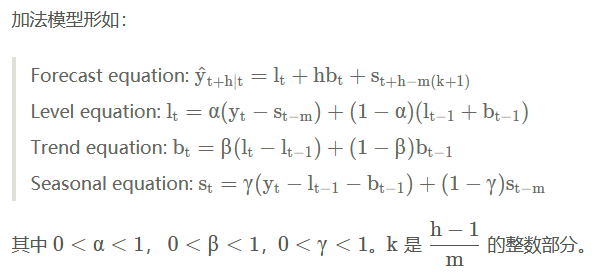##### 4.1.2 Holt-Winters’ multiplicative method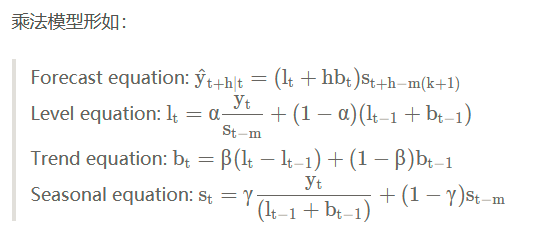##### 4.1.3 Holt-Winters’ damped method

Holt-Winters’ 模型的趋势部分同样可以引入阻尼系数 ϕ\phiϕ，这里不再赘述。

#### 4.2 代码展示

import numpy as np
import pandas as pd
import matplotlib.pyplot as plt

def holtwinters():
from statsmodels.tsa.holtwinters import ExponentialSmoothing

number = 100
x3 = np.round(np.linspace(0, 4 * np.pi, number))
y3 = pd.Series(20 + 0.1 * np.multiply(x3, x3) + 8 * np.cos(2 * x3) + 2 * np.random.randn(number))
# ets3 = ExponentialSmoothing(y3, trend='mul', seasonal='mul', seasonal_periods=25)
ets3 = ExponentialSmoothing(y3, trend='mul', seasonal='mul', damped_trend=True, seasonal_periods=25)
r3 = ets3.fit()
pred3 = r3.predict(start=len(y3), end=len(y3) + len(y3) // 2)

pd.DataFrame({
'origin': y3,
'fitted': r3.fittedvalues,
'pred': pred3
}).plot(legend=True)
plt.savefig('holtwinters_mul_damped.png')

holtwinters()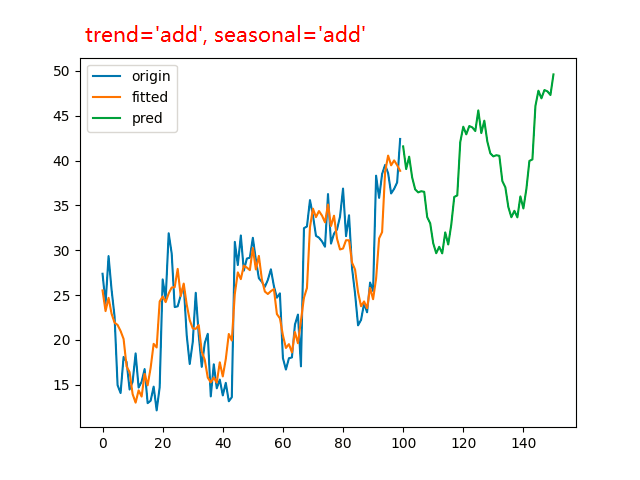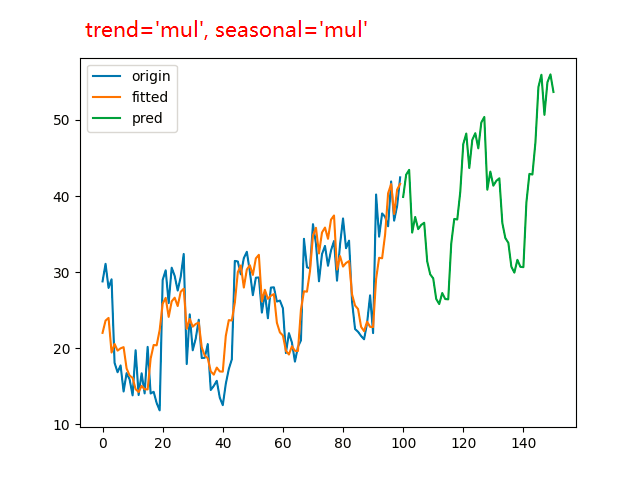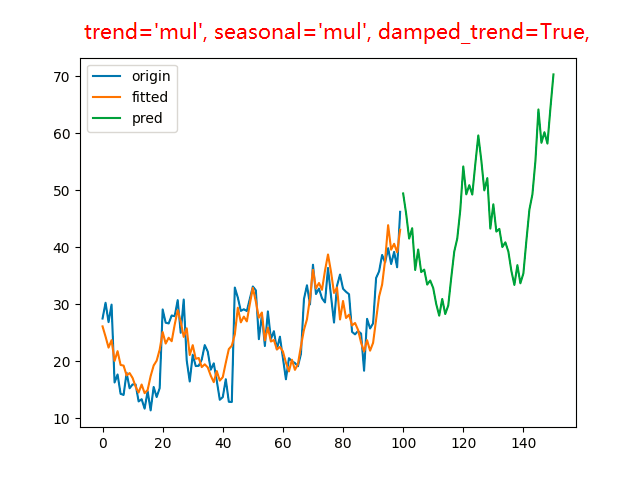#### 4.3 参数介绍

Holt Winter's Exponential Smoothing

Parameters
----------
endog : array_like
The time series to model.
Type of trend component.
damped_trend : bool, optional
Should the trend component be damped.
Type of seasonal component.
seasonal_periods : int, optional
The number of periods in a complete seasonal cycle, e.g., 4 for
quarterly data or 7 for daily data with a weekly cycle.
initialization_method : str, optional
Method for initialize the recursions. One of:

* None
* 'estimated'
* 'heuristic'
* 'legacy-heuristic'
* 'known'

None defaults to the pre-0.12 behavior where initial values
are passed as part of fit. If any of the other values are
passed, then the initial values must also be set when constructing
the model. If 'known' initialization is used, then initial_level
must be passed, as well as initial_trend and initial_seasonal if
applicable. Default is 'estimated'. "legacy-heuristic" uses the same
values that were used in statsmodels 0.11 and earlier.
initial_level : float, optional
The initial level component. Required if estimation method is "known".
If set using either "estimated" or "heuristic" this value is used.
This allows one or more of the initial values to be set while
deferring to the heuristic for others or estimating the unset
parameters.
initial_trend : float, optional
The initial trend component. Required if estimation method is "known".
If set using either "estimated" or "heuristic" this value is used.
This allows one or more of the initial values to be set while
deferring to the heuristic for others or estimating the unset
parameters.
initial_seasonal : array_like, optional
The initial seasonal component. An array of length seasonal
or length seasonal - 1 (in which case the last initial value
is computed to make the average effect zero). Only used if
initialization is 'known'. Required if estimation method is "known".
If set using either "estimated" or "heuristic" this value is used.
This allows one or more of the initial values to be set while
deferring to the heuristic for others or estimating the unset
parameters.
use_boxcox : {True, False, 'log', float}, optional
Should the Box-Cox transform be applied to the data first? If 'log'
then apply the log. If float then use the value as lambda.
bounds : dict[str, tuple[float, float]], optional
An dictionary containing bounds for the parameters in the model,
excluding the initial values if estimated. The keys of the dictionary
are the variable names, e.g., smoothing_level or initial_slope.
The initial seasonal variables are labeled initial_seasonal.<j>
for j=0,...,m-1 where m is the number of period in a full season.
Use None to indicate a non-binding constraint, e.g., (0, None)
constrains a parameter to be non-negative.
dates : array_like of datetime, optional
An array-like object of datetime objects. If a Pandas object is given
for endog, it is assumed to have a DateIndex.
freq : str, optional
The frequency of the time-series. A Pandas offset or 'B', 'D', 'W',
'M', 'A', or 'Q'. This is optional if dates are given.
missing : str
Available options are 'none', 'drop', and 'raise'. If 'none', no nan
checking is done. If 'drop', any observations with nans are dropped.
If 'raise', an error is raised. Default is 'none'.


霍尔特-温特指数平滑

参数

----------

endog:数组类型
要建模的时间序列。

趋势组件的类型。

阻尼趋势：bool，可选
趋势成分应该被抑制。

季节性成分的类型。

季节性周期：int，可选
一个完整的季节性周期中的周期数，例如，季度数据为4，周周期的日数据为7。

初始化方法：str，可选
方法初始化递归。什么之中的一个：

*没有
*'估计'
*“启发式”
*'传统启发式'
*'已知'

None默认为0.12之前的行为，其中初始值作为“fit”的一部分传递。如果传递了任何其他值，那么在构建模型时也必须设置初始值。如果使用“known”初始化，则必须传递“initial_level”，以及“initial_trend”和“initial_seasional”（如果适用）。默认值为“估计”“遗留启发式”使用与statsmodels 0.11和更早版本中使用的值相同的值。

initial_level : float, optional 可选
初始级别组件。如果估算方法为“已知”，则需要。如果使用“估计”或“启发式”设置，则使用此值。这允许设置一个或多个初始值，同时遵从其他启发式或估计未设置的参数。

initial_trend : float, optional
初始趋势成分。如果估算方法为“已知”，则需要。如果使用“估计”或“启发式”设置，则使用此值。这允许设置一个或多个初始值，同时遵从其他启发式或估计未设置的参数。

initial_seasonal : array_like, optional
最初的季节性成分。长度为“seasional”或长度为“seasional-1”的数组（在这种情况下，计算最后一个初始值以使平均效果为零）。仅在初始化为“已知”时使用。如果估算方法为“已知”，则需要。如果使用“估计”或“启发式”设置，则使用此值。这允许设置一个或多个初始值，同时遵从其他启发式或估计未设置的参数。

use_boxcox : {True, False, 'log', float}, optional
是否应该首先对数据应用Box-Cox变换？如果是'log'，则应用日志。如果是float，则使用lambda值。

bounds : dict[str, tuple[float, float]], optional
一个字典，包含模型中参数的界限，如果估计，则不包括初始值。字典的键是变量名，例如，smoothing_level 或 initial_slope。初始季节变量被标记为initial_seasonal。<j> for j=0,...,m-1，其中m是整个季节的周期数。使用None表示非绑定约束，例如，（0，None）将参数约束为非负。

dates : array_like of datetime, optional
datetime对象的类似数组的对象。如果为endog指定了Pandas对象，则假定该对象具有DateIndex。

freq : str, optional
时间序列的频率。A或“B”、“D”、“W”、“M”、“A”或“Q”。如果给出了日期，这是可选的。

missing : str
可用选项有 'none', 'drop', and 'raise' 。如果'none'，则不进行nan检查。如果是“drop”，则任何与nan有关的观测都将被丢弃。如果“raise”，则会引发错误。默认值为'none'。

Fit the model

Parameters
----------
smoothing_level : float, optional
The alpha value of the simple exponential smoothing, if the value
is set then this value will be used as the value.
smoothing_trend :  float, optional
The beta value of the Holt's trend method, if the value is
set then this value will be used as the value.
smoothing_seasonal : float, optional
The gamma value of the holt winters seasonal method, if the value
is set then this value will be used as the value.
damping_trend : float, optional
The phi value of the damped method, if the value is
set then this value will be used as the value.
optimized : bool, optional
Estimate model parameters by maximizing the log-likelihood.
remove_bias : bool, optional
Remove bias from forecast values and fitted values by enforcing
that the average residual is equal to zero.
start_params : array_like, optional
Starting values to used when optimizing the fit.  If not provided,
starting values are determined using a combination of grid search
and reasonable values based on the initial values of the data. See
the notes for the structure of the model parameters.
method : str, default "L-BFGS-B"
The minimizer used. Valid options are "L-BFGS-B" , "TNC",
"SLSQP" (default), "Powell", "trust-constr", "basinhopping" (also
"bh") and "least_squares" (also "ls"). basinhopping tries multiple
starting values in an attempt to find a global minimizer in
non-convex problems, and so is slower than the others.
minimize_kwargs : dict[str, Any]
A dictionary of keyword arguments passed to SciPy's minimize
function if method is one of "L-BFGS-B", "TNC",
"SLSQP", "Powell", or "trust-constr", or SciPy's basinhopping
or least_squares functions. The valid keywords are optimizer
specific. Consult SciPy's documentation for the full set of
options.
use_brute : bool, optional
Search for good starting values using a brute force (grid)
optimizer. If False, a naive set of starting values is used.
use_boxcox : {True, False, 'log', float}, optional
Should the Box-Cox transform be applied to the data first? If 'log'
then apply the log. If float then use the value as lambda.

.. deprecated:: 0.12

Set use_boxcox when constructing the model

use_basinhopping : bool, optional
Deprecated. Using Basin Hopping optimizer to find optimal values.
Use method instead.

.. deprecated:: 0.12

Use method instead.

initial_level : float, optional
Value to use when initializing the fitted level.

.. deprecated:: 0.12

Set initial_level when constructing the model

initial_trend : float, optional
Value to use when initializing the fitted trend.

.. deprecated:: 0.12

Set initial_trend when constructing the model
or set initialization_method.

Returns
-------
HoltWintersResults
See statsmodels.tsa.holtwinters.HoltWintersResults.


### 5、参数优化和模型选择理论——AIC BIC

参数优化的方法是最小化误差平方和或最大化似然函数。模型选择可以根据信息量准则，常用的有 AIC 和 BIC等。

（1）AIC 即 Akaike information criterion， 定义为
A I C = 2 k − 2 l n L ( θ ) AIC = 2k-2lnL(\theta)
其中 L(θ) 是似然函数， k是参数数量。用 AIC 选择模型时要求似然函数大，同时对参数数量作了惩罚，在似然函数相近的情况下选择复杂度低的模型。

（2）BIC 即 Bayesian information criterion，定义为
B I C = k l n k − 2 l n L ( θ ) BIC = klnk-2lnL(\theta)
其中 n n 是样本数量。当 n > e 2 ≈ 7.4 n>e^2≈7.4 时， k l n ⁡ n > 2 k kln⁡n>2k ，因此当样本量较大时 BIC 对模型复杂度的惩罚比 AIC 更严厉。

### 6、 与 ARIMA 的关系

线性的指数平滑方法可以看作是 ARIMA 的特例。例如简单指数平滑等价于 ARIMA(0, 1, 1)，Holt’s linear trend method 等价于 ARIMA(0, 2, 2)，而 Damped trend methods 等价于 ARIMA(1, 1, 2) 等。

数学推理过程如下：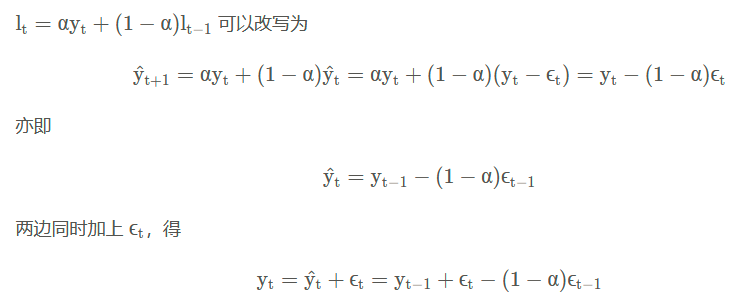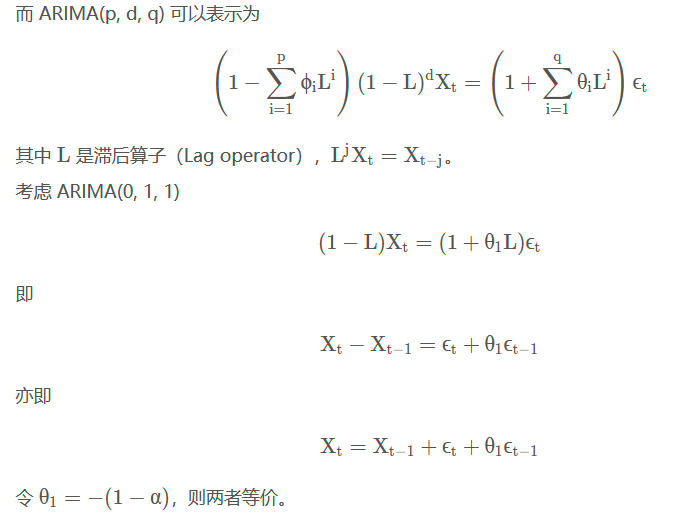非线性的指数平滑方法则没有对应的 ARIMA 表示。【这句话的含义还未理解。】

展开全文python r语言
• matlab中可用于预测的三次指数平滑法，针对有二次趋势的数据
• 指数平滑法相比于移动平均法，它是一种特殊的加权平均方法。简单移动平均法用的是算术平均数，近期数据对预测值的影响比远期数据要大一些，而且越近的数据影响越大。指数平滑法正是考虑了这一点，并将其权值按指数...
• 背景指数平滑是在 20 世纪 50 年代后期提出的，并激发了一些十分成功的预测方法。使用指数平滑方法生成的预测是过去观测值的加权平均值，并且随着过去观测值离预测值距离的增大，权重呈指数型衰...python 机器学习 人工智能 深度学习
• CSDN同步参考链接指数平滑法说起来很简单，不只是几个周期的平均值，但是您知道在Eviews中进行指数平滑时如何确定1、的初始值吗？2、可以根据需要更改确定初始值的方法吗？3、期末水平：在Eviews获得的结果中意味着...
• 指数平滑方法说起来感觉挺简单的，不就是几期求均值吗，但是你知道在Eviews里做指数平滑模型的时候，1、他的初始值是如何确定的吗？2、初始值的确定方法可以按照我们想的去改变吗？ 3、Eviews得到结果中的 End of ...
• 原文地址：... 指数平滑法（Exponential Smoothing，ES） 目录 1什么是指数平滑法 2指数平滑法的基本公式 3指数平滑的预测公式 3.1（一） 一次指数平滑预测 ...
• 该文档为原创文档，内容是一次二次三次指数平滑方法的3份数据和对应的操作，对我们深刻理解指数平滑有很大帮助哦。
•时间序列预测
•时间序列预测
• 由此可以得到最优的平滑系数，这里可以引入线性规划的思想来求得最优解但是：python没有线性规划的包，所以就没有细致的代码写出来了，不过经过手动计算尝试这样子是可行的在python3下编程，一次指数平滑代码为：1 ...
• 简单平滑方法中“下一期预测值等于当期真实值与当期预测值的加权值”。如果“下一期预测值等于当期真实值与上一期预测值的加权值”，则将损失掉1/2的真实值信息；即当等号右边进行加权的预测值项比真实值项滞后一期...时间序列分析
• 然而，在许多预测问题中，经典的方法，如SARIMA和指数平滑法，容易优于更复杂的方法。因此，在探索更先进的方法之前，既要了解经典时间序列预测方法的工作原理，又要对其进行评估。本文介绍了时间序列预测的原始和...机器学习 深度学习 时间序列预测 ARIMA
•时间序列预测
• 上文我们使用LOF-ICAD方法实现了时序数据的异常检测, 这次我们介绍一种更为常见的方法——-指数平滑. 指数平滑方法, 其原理就是通过拟合出一个近似的模型来对未来进行预测, 我们可以通过这个预测来和实际的值进行...异常检测 java 算法 时序数据 数据挖掘
• java代码实现指数平滑算法，其中包括一次，二次，三次
• 用于比较不同权重系数的一次指数平滑优劣的通用程序。待分析的时间序列可根据喜好选择通过input命令在命令窗口输入，或直接在程序中给出。
• 3.2 时间序列的指数平滑预测法指数平滑法(Expinential smoothing method)的思想也是对时间序列进行修匀以消除不规则和随机的扰动。该方法是建立在如下基础上的加权平均法：即认为时间序列中的近期数据对未来值的影响...
• Matlab实现三次指数平滑算法Matlab实现三次指数平滑算法Matlab实现三次指数平滑算法Matlab实现三次指数平滑算法Matlab实现三次指数平滑算法
• 利用EXCEL软件中的指数平滑分析工具对贵州省2000年至2010年11年的煤炭产量数据进行分析,分别测算几个不同平滑指数下的一次指数平滑结果,根据偏差平方最优原理选择合适的平滑指数,再通过二次指数平滑,得出贵州省煤炭...
• 指数平滑用来做时间序列预测，数据是单个时间序列，输入数据格式为二维数据， 一维数据，只需定义一个一行多列的数组即可
• 指数平滑算法，包括一次指数，二次指数，三次指数，且有注释说明怎么使用
• ## 简单指数平滑

千次阅读 2018-03-24 15:51:38
简单指数平滑 简单指数平滑法（SES）适用于预测没有趋势和季节性的模型。 y^T+1|T=αyT+α(1−α)yT−1+α(1−α)2yT−2+⋯,y^T+1|T=αyT+α(1−α)yT−1+α(1−α)2yT−2+⋯,\hat{y}_{T+1|T} = \alpha y_T + \...
• 修正： 式④中第一项，(Lt / Lt-1)应改为(Lt - Lt-1)，因为该项表示由level项算出的从下一点与当前点间的斜率，应用减法而不是除法。时间序列预测...